# ISEE Lower Level Math : How to find the area of a triangle

## Example Questions

### Example Question #1231 : Isee Lower Level (Grades 5 6) Mathematics Achievement

What is the area of a triangle for which the base is 9 inches and the height is 12 inches?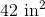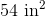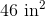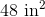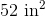Explanation:

The area of a triangle is found by multiplying the base times the height, divided by 2.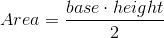Given that the base is 9 inches and the height is 12 inches, plugging these numbers into the equation gives us the following: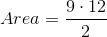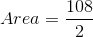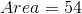### Example Question #11 : How To Find The Area Of A Triangle

If a triangle has a height of 2 inches and a base that is 3 times as long, what is the area?Explanation:

The area of a triangle is found by multiplying the base times the height, divided by 2.

Given that the height is 2 inches, and the base is 3 times that of the height, the base is 6 inches.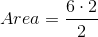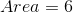### Example Question #301 : Geometry

If the area of a triangle is 15 feet and the height is 5 feet, what is the length of the base?Explanation:

The area of a triangle is found by multiplying the base times the height, divided by 2.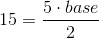Each side of the equation should be multiplied by 2.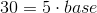Each side should be divided by 5.

Therefore, the base has a value of 6.

### Example Question #11 : How To Find The Area Of A Triangle

A triangle has a height of 6 inches and a base of 3 inches. What is the area, in square inches?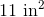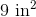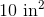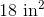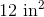Explanation:

The area of a triangle is found by multiplying the base times the height, divided by 2.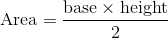Here, the base is 3 inches and the height is 6 inches. When plugging this information into the formula, we get: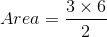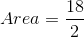This fraction reduces to 9, the correct answer.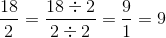### Example Question #11 : How To Find The Area Of A Triangle

Find the area of a triangle whose base isand height is.Explanation:

To solve, simply use the formula for the area of a triangle. Thus,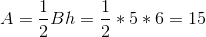### Example Question #1241 : Isee Lower Level (Grades 5 6) Mathematics Achievement

If you have a right triangle with a height of 6 inches, a base of 8 inches, and a hypotenuse of 10 inches, what is its area?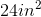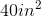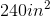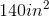Explanation:

If you have a right triangle with a height of 6 inches, a base of 8 inches, and a hypotenuse of 10 inches, what is its area?

To find area of a triangle, we use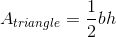So, plug in our base and height measurements to get our answer: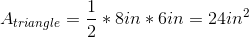Coincidentally, the same as our perimeter

### Example Question #11 : Triangles

How much area does a triangular garden have with a base ofand a height of?Explanation:

The area of a triangle is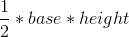so given that the base is 5 and the height is 6, the area equation becomes,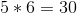and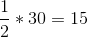.

### Example Question #11 : Triangles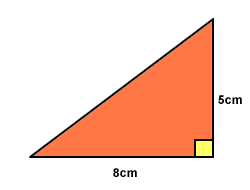If the formula for the area of a triangle is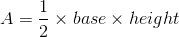,

what is the area of this triangle?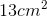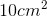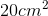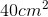Explanation:

Using the formula: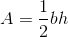where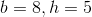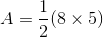Since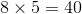the equation becomes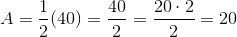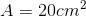Note: Answer will always be represented with the measurement to the second power when calculating the area of a triangle.

### Example Question #12 : How To Find The Area Of A Triangle

Find the area of a triangle with a height of 12in and a base that is half the height.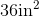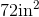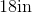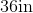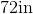Explanation:

The formula to find the area of a triangle is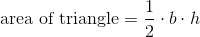where b is the base and h is the height of the triangle.

We know the height of the triangle is 12in.  We know the base is half the height, so the base is 6in.  Now, we can substitute.  We get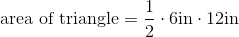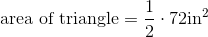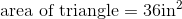### Example Question #11 : How To Find The Area Of A Triangle

The base of a triangle isinches, and the height of the triangle isinches.  What is the area of the triangle?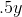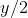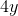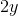Explanation:

To find the area of a triangle, multiply the base by the height, and divide by two.  The best answer is: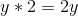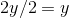### All ISEE Lower Level Math Resources